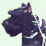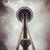# Calculate Geometric Mean

3056
8
02-11-2013 12:31 PMNew Contributor
Hello!

Does anyone happen to know if it's possible to calculate geometric mean as a summary statistic in ArcGIS 10.1?

Thanks,

Amy
Tags (3)
8 RepliesRegular Contributor
Try the Mean Center toolNew Contributor
Try the Mean Center tool

Thanks for the suggestion! Do you know if I would be able to use the Mean Center tool to calculate geometric mean (as opposed to arithmetic mean) for a field that contains numeric values (such as integers or floats), rather than the shape's geographic coordinates?

Take care,

AmyRegular Contributor
You can input a Weight Field to the Mean Center tool -- is this what you mean?
For more information, take a look at the "how it works" doc.New Contributor
You can input a Weight Field to the Mean Center tool -- is this what you mean?
For more information, take a look at the "how it works" doc.

Although the data I'm working with is spatial in nature, the statistical calculation (described here: http://en.wikipedia.org/wiki/Geometric_mean) would not require using a feature's spatial information. For example, if performing a Dissolve, I would like to use the geometric mean (as opposed to arithmetic) as a merge rule.Regular Contributor
Ah...  that geometric mean!
Not that I know of.  I'll have to do some checking.  For sure Dissolve doesn't have the option.New Contributor
Ah...  that geometric mean!
Not that I know of.  I'll have to do some checking.  For sure Dissolve doesn't have the option.

Thanks! I'm sure it could be done with some Python scripting, but it would certainly be quicker and easier if a geoprocessing tool such as Dissolve provided that option. I thought it couldn't hurt to ask. :)Occasional Contributor III

Hi Amy - Did you ever find a way to do this in ArcMap?New Contributor

Hi! We ended up using Python scripting outside of ArcMap and arcpy.